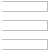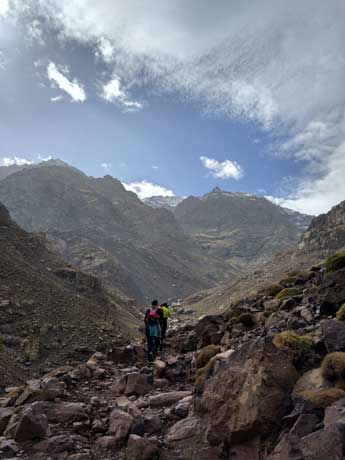TOPIC 4: ENERGY

# 4.2 Energy efficiency

Walking up hill is hard work. (Figure 1). The walkers here are transferring stored chemical energy, originally from food, into gravitational energy at the top of the hill.

In an ideal world, all of the energy we transfer from chemical energy in our bodies would end up as gravitational energy. However, we all know that this exercise would make us hot. Some of the energy has been transferred into thermal energy, which we then struggle to dissipate into the air by sweating and other processes.Figure 1. Walking up a steep hill

This loss of energy, wasted as thermal energy, can be represented in a special type of flow diagram, as shown in figure 2 below:Figure 2. A sankey diagram for walking up a steep hill

This type of diagram is called a sankey diagram. It is basically showing how energy is transferred from one form to another. The arrows are drawn to scale, to represent the quantity of energy transferred into a useful form, and also to represent how much energy is wasted - usually dissipated as thermal energy in the surroundings.

## Efficiency

Energy is measured in joules (J), so in the sankey diagram in figure 2 we start with 200 kJ (200 000 joules) of chemical energy. However, our bodies are not 100% efficient, as discussed above. What percentage of our chemical energy is successfully converted into useful gravitational energy? To find this out, we need to do some simple maths - what percentage of 200 kJ is 80 kJ? This answer gives us the efficiency of the transfer as a percentage.

There is a useful standard formula to help you work out the efficiency of any process that does work by transferring energy:

 efficiency = useful energy output x 100% energy input
Learn this formula!

Example:
What is the efficiency of the climber shown in figure 1 above? Use data from the sankey diagram shown in figure 2.

In figure 2, the useful energy output is 80 kJ (80 000 J), and the input is 200 kJ (200 000 J). Using the formula above gives:

 efficiency = 80 000 x 100% 200 000

So the efficiency is 40 %.

Note that it is possible to use measurements for power input and power output in the same way, and some sankey diagrams give readings in 'watts' to show the power input and output, rather than joules.

To practise this formula, have a go at the following questions based on the sankey diagram shown below.

Questions:

1. A small toy car uses a spring inside to drive the car. The spring can be wound up using a key, and then the car released.

This sankey diagram shows the energy transfers that take place. Some labels are missing from the diagram.• a) What form of energy is stored in the spring at the start?
• b) What form of energy store does the car have once it is released?
• c) How much energy is dissipated as heat into the surroundings?
• d) State the formula for efficiency.
• e) Calculate the efficiency of the toy car.

a) The spring stores elastic energy.

b) Once the car is released, the energy stored into the spring as elastic energy is transferred to kinetic energy.

c) The energy lost as wasted thermal energy is dissipated into the surrounds. The missing value is 24 J - 18 J = 6 J

d) The formula is:

 efficiency = useful energy output x 100% energy input

e) Substituting in values from the diagram gives:

 efficiency = 18 x 100% 24

Efficiency = 75 %

Now test your understanding using these quick, 10 minute questions on this topic from Grade Gorilla:

(opens in a new tab)